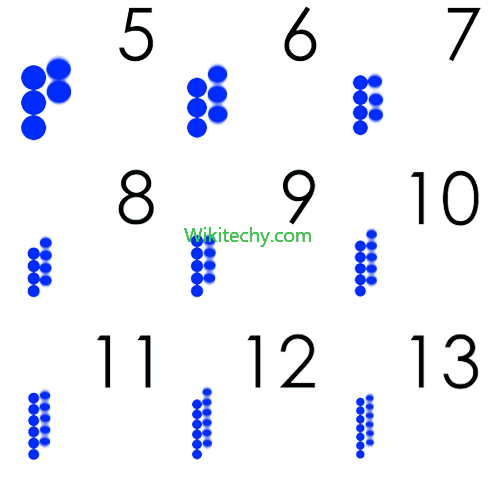# C# Prime Number - c# - c# tutorial - c# net

## How to write Prime number Program in C# ?

• Prime number is a number that is greater than 1 and divided by 1 or itself.
• In other words, prime numbers can't be divided by other numbers than itself or 1.
• For example- 2, 3, 5, 7, 11, 13, 17, 19, 23.... are the prime numbers.
• Let's see the prime number program in C#.
• In this C# program, we will take an input from the user and check whether the number is prime or not.C# Prime Number